KDnuggets Home » News » 2018 » Feb » Tutorials, Overviews » A Tour of The Top 10 Algorithms for Machine Learning Newbies ( 18:n09 )

#A Tour of The Top 10 Algorithms for Machine Learning Newbies

For machine learning newbies who are eager to understand the basic of machine learning, here is a quick tour on the top 10 machine learning algorithms used by data scientists.comments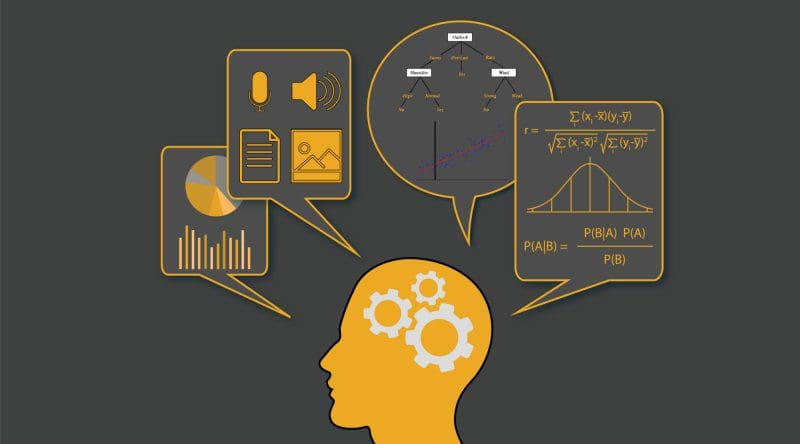In machine learning, there’s something called the “No Free Lunch” theorem. In a nutshell, it states that no one algorithm works best for every problem, and it’s especially relevant for supervised learning (i.e. predictive modeling).

For example, you can’t say that neural networks are always better than decision trees or vice-versa. There are many factors at play, such as the size and structure of your dataset.

As a result, you should try many different algorithms for your problem, while using a hold-out “test set” of data to evaluate performance and select the winner.

Of course, the algorithms you try must be appropriate for your problem, which is where picking the right machine learning task comes in. As an analogy, if you need to clean your house, you might use a vacuum, a broom, or a mop, but you wouldn’t bust out a shovel and start digging.

The Big Principle

However, there is a common principle that underlies all supervised machine learning algorithms for predictive modeling.

Machine learning algorithms are described as learning a target function (f) that best maps input variables (X) to an output variable (Y): Y = f(X)

This is a general learning task where we would like to make predictions in the future (Y) given new examples of input variables (X). We don’t know what the function (f) looks like or its form. If we did, we would use it directly and we would not need to learn it from data using machine learning algorithms.

The most common type of machine learning is to learn the mapping Y = f(X) to make predictions of Y for new X. This is called predictive modeling or predictive analytics and our goal is to make the most accurate predictions possible.

For machine learning newbies who are eager to understand the basic of machine learning, here is a quick tour on the top 10 machine learning algorithms used by data scientists.

### 1 — Linear Regression

Linear regression is perhaps one of the most well-known and well-understood algorithms in statistics and machine learning.

Predictive modeling is primarily concerned with minimizing the error of a model or making the most accurate predictions possible, at the expense of explainability. We will borrow, reuse and steal algorithms from many different fields, including statistics and use them towards these ends.

The representation of linear regression is an equation that describes a line that best fits the relationship between the input variables (x) and the output variables (y), by finding specific weightings for the input variables called coefficients (B).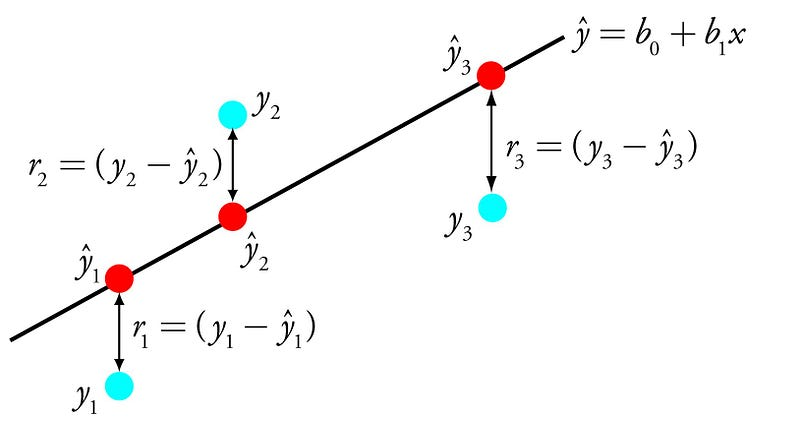Linear Regression

For example: y = B0 + B1 * x

We will predict y given the input x and the goal of the linear regression learning algorithm is to find the values for the coefficients B0 and B1.

Different techniques can be used to learn the linear regression model from data, such as a linear algebra solution for ordinary least squares and gradient descent optimization.

Linear regression has been around for more than 200 years and has been extensively studied. Some good rules of thumb when using this technique are to remove variables that are very similar (correlated) and to remove noise from your data, if possible. It is a fast and simple technique and good first algorithm to try.

### 2 — Logistic Regression

Logistic regression is another technique borrowed by machine learning from the field of statistics. It is the go-to method for binary classification problems (problems with two class values).

Logistic regression is like linear regression in that the goal is to find the values for the coefficients that weight each input variable. Unlike linear regression, the prediction for the output is transformed using a non-linear function called the logistic function.

The logistic function looks like a big S and will transform any value into the range 0 to 1. This is useful because we can apply a rule to the output of the logistic function to snap values to 0 and 1 (e.g. IF less than 0.5 then output 1) and predict a class value.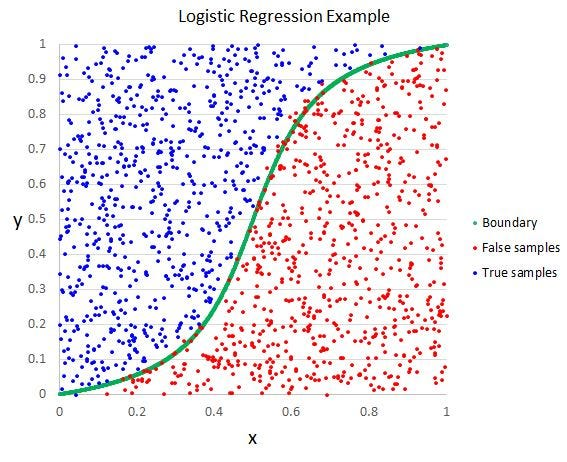Logistic Regression

Because of the way that the model is learned, the predictions made by logistic regression can also be used as the probability of a given data instance belonging to class 0 or class 1. This can be useful for problems where you need to give more rationale for a prediction.

Like linear regression, logistic regression does work better when you remove attributes that are unrelated to the output variable as well as attributes that are very similar (correlated) to each other. It’s a fast model to learn and effective on binary classification problems.

### 3 — Linear Discriminant Analysis

Logistic Regression is a classification algorithm traditionally limited to only two-class classification problems. If you have more than two classes then the Linear Discriminant Analysis algorithm is the preferred linear classification technique.

The representation of LDA is pretty straight forward. It consists of statistical properties of your data, calculated for each class. For a single input variable this includes:

1. The mean value for each class.
2. The variance calculated across all classes.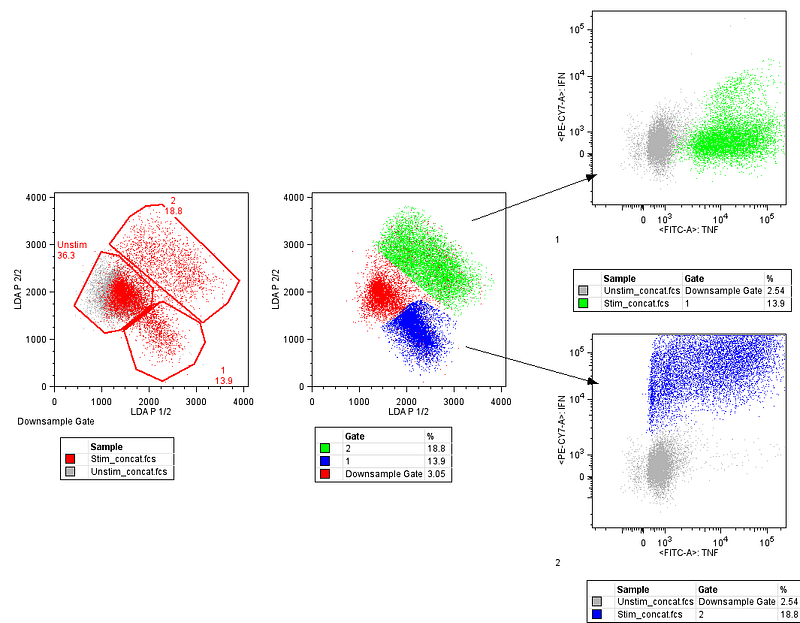Linear Discriminant Analysis

Predictions are made by calculating a discriminate value for each class and making a prediction for the class with the largest value. The technique assumes that the data has a Gaussian distribution (bell curve), so it is a good idea to remove outliers from your data before hand. It’s a simple and powerful method for classification predictive modeling problems.

### 4 — Classification and Regression Trees

Decision Trees are an important type of algorithm for predictive modeling machine learning.

The representation of the decision tree model is a binary tree. This is your binary tree from algorithms and data structures, nothing too fancy. Each node represents a single input variable (x) and a split point on that variable (assuming the variable is numeric).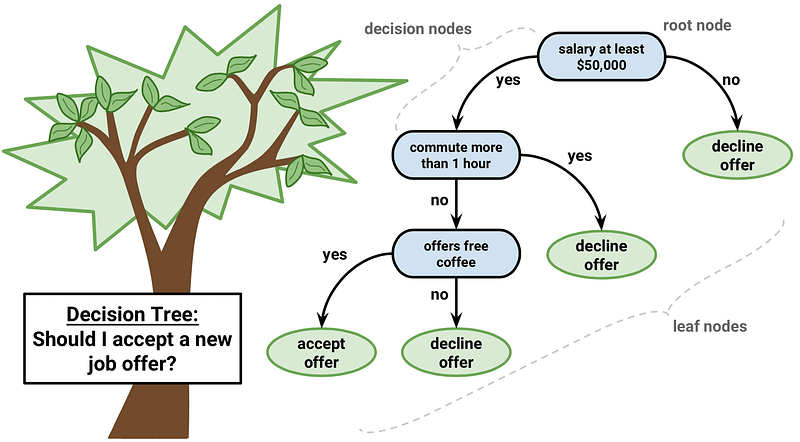Decision Tree

The leaf nodes of the tree contain an output variable (y) which is used to make a prediction. Predictions are made by walking the splits of the tree until arriving at a leaf node and output the class value at that leaf node.

Trees are fast to learn and very fast for making predictions. They are also often accurate for a broad range of problems and do not require any special preparation for your data.

### 5 — Naive Bayes

Naive Bayes is a simple but surprisingly powerful algorithm for predictive modeling.

The model is comprised of two types of probabilities that can be calculated directly from your training data: 1) The probability of each class; and 2) The conditional probability for each class given each x value. Once calculated, the probability model can be used to make predictions for new data using Bayes Theorem. When your data is real-valued it is common to assume a Gaussian distribution (bell curve) so that you can easily estimate these probabilities.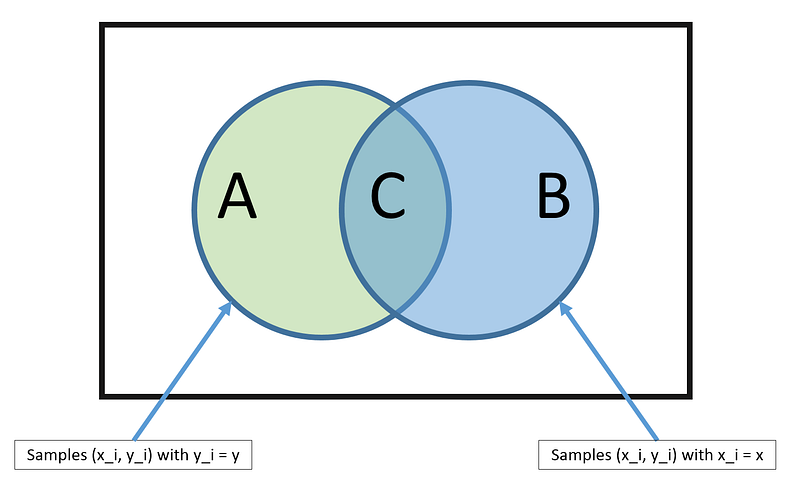Bayes Theorem

Naive Bayes is called naive because it assumes that each input variable is independent. This is a strong assumption and unrealistic for real data, nevertheless, the technique is very effective on a large range of complex problems.Get KDnuggets, a leading newsletter on AI, Data Science, and Machine Learning